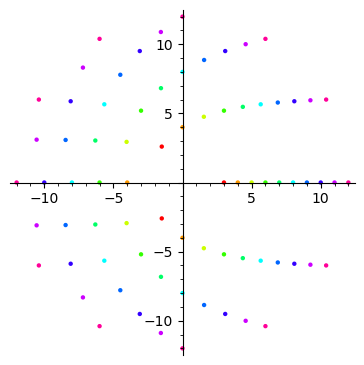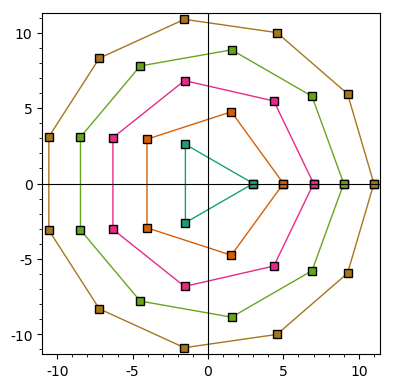# Automatically adding colors to multiple list_plot graphics

Is there a way to automatically color different plots?

For example, I'm trying to plot complex points using list_plot, and the points are all stored in a list of lists, each of which I would like to be a different color. So, in order to plot these points, I have

list=[list1,list2,...]
plot = sum(list_plot(i) for i in list)


I'm wondering if there's any (somewhat simple) way to add different colors corresponding to each list in an iterable fashion, rather than typing out

plot=list_plot([i for i in list1], rgbcolor='red')+list_plot([i for i in list2], rgbcolor='orange')+...

edit retag close merge delete

Sort by » oldest newest most voted

One way would be to use the hue.

Example:

sage: tau = 2*pi
sage: ngon = lambda n: [(n*cos(k*tau/n), n*sin(k*tau/n)) for k in range(n)]
sage: point_lists = [ngon(n) for n in range(3, 13)]
sage: n = len(point_lists)
sage: G = sum(list_plot(point_list, hue=k/n) for k, point_list in enumerate(point_lists))
sage: G.show(aspect_ratio=1)moreInstead of the hue, as suggested by @slelievre, you could use colors extracted from a color map. For example, borrowing and slightly modifying slelievre's code, you could write:

tau = 2*pi
ngon = lambda n: [(n*cos(k*tau/n), n*sin(k*tau/n)) for k in range(n+1)]
point_lists = [ngon(n) for n in range(3, 13, 2)]
n = len(point_lists)
ops = dict(plotjoined=True, markeredgecolor="black", marker="s")
col = lambda c: colormaps.Dark2(c)[0:3]
G = sum(list_plot(point_list, color=col(k/n), **ops)
for k, point_list in enumerate(point_lists))
G.show(aspect_ratio=1,frame=True)You could replace colormaps.Dark2 by, say, colormaps.jet, colormaps.Blues, etc. For a complete list of predefined color maps, type list(colormaps).

more